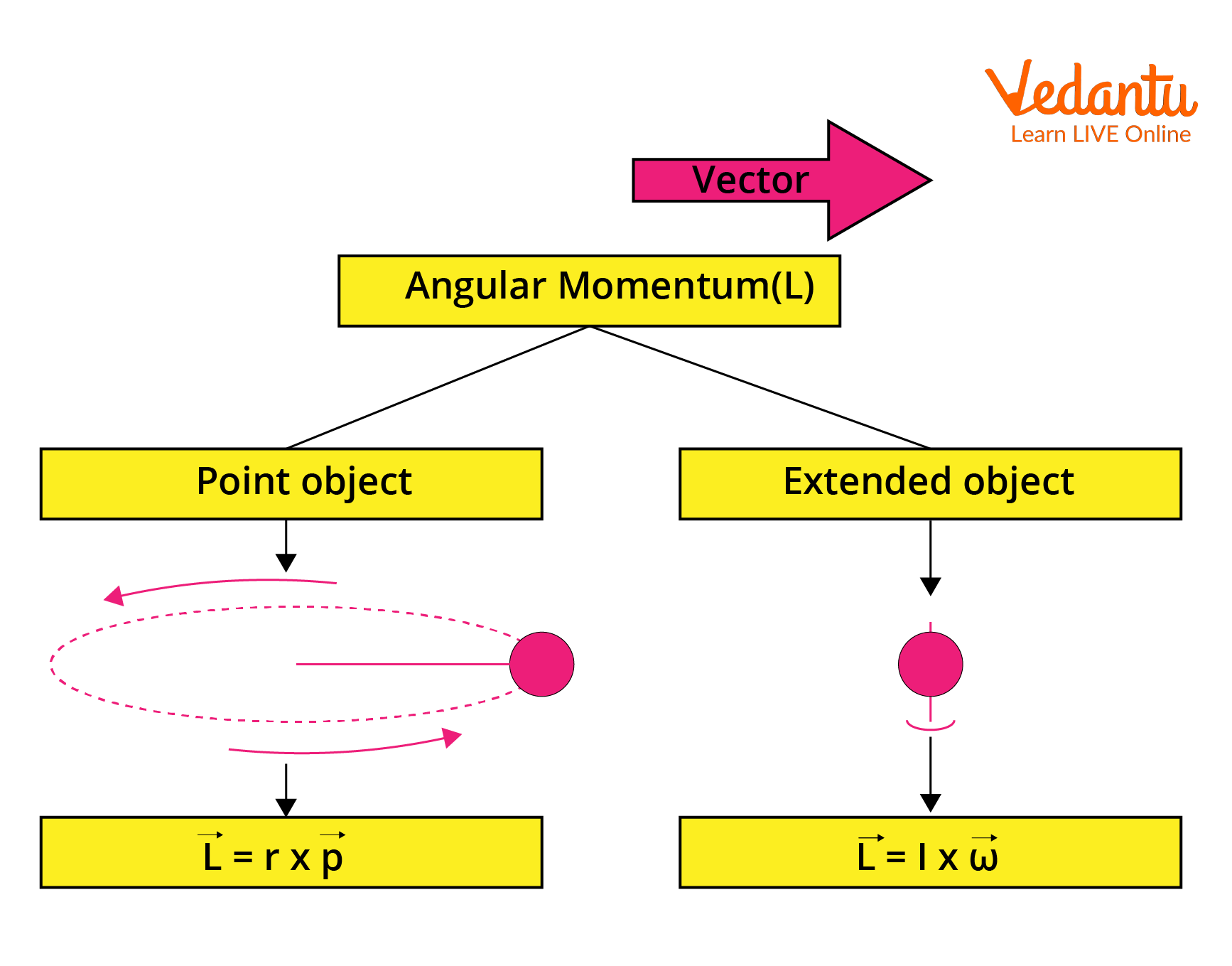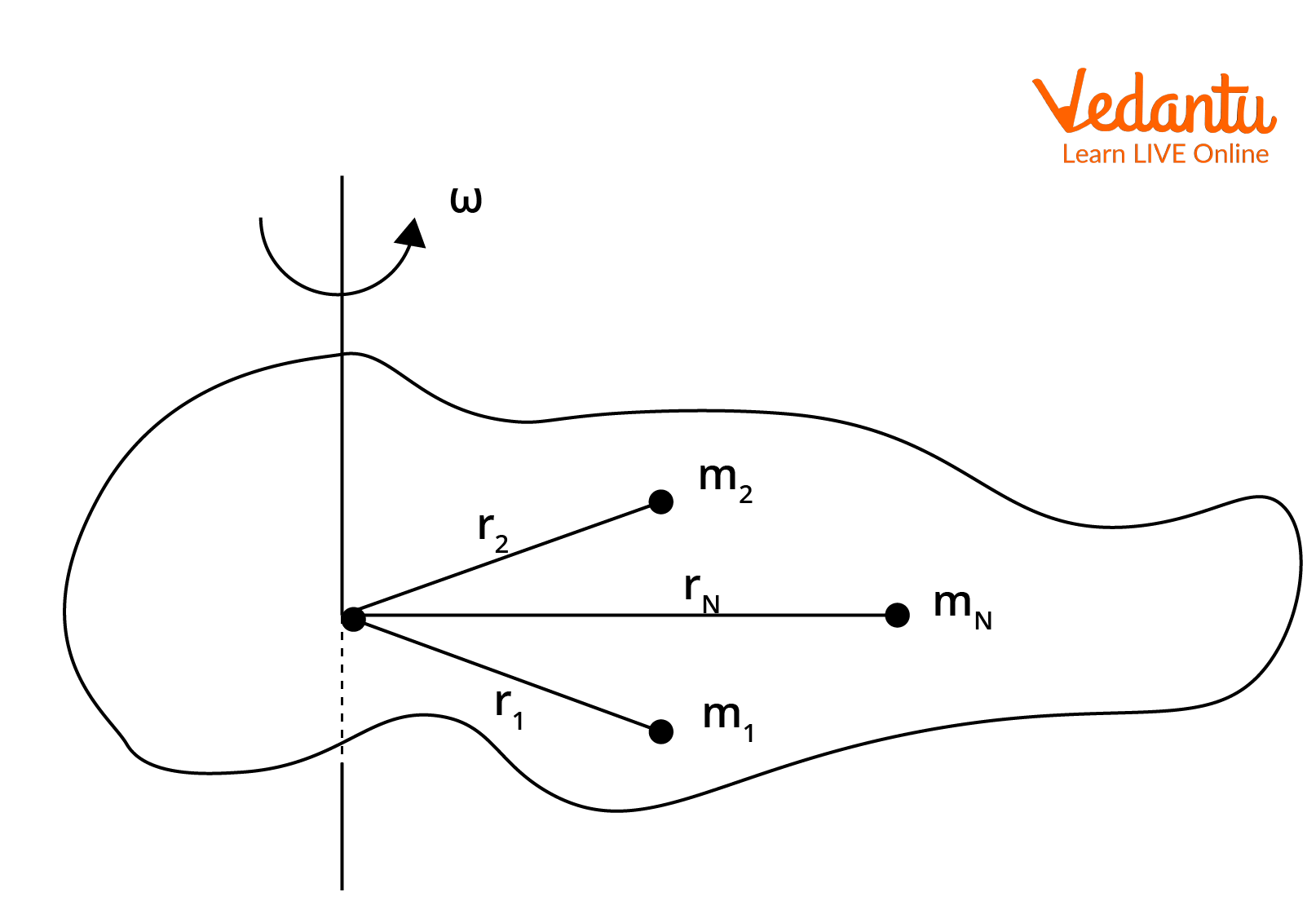Courses
Courses for Kids
Free study material
Free LIVE classes
More

# Angular Momentum of Rotating Body - Important Topic for JEELIVE
Join Vedantu’s FREE Mastercalss

## What is Momentum?

Before moving on to angular momentum, it is important to have an idea about momentum. Momentum basically refers to the quantity of motion that any body in motion has. It is obvious that momentum is defined for objects in motion. This means that if an object is in motion, it has a certain momentum.  We all have heard the word momentum being used in our everyday life. In sports, the word "momentum" is frequently used. A squad that is moving forward and has momentum will be difficult to stop. A team that is genuinely moving forward and gaining momentum will be challenging to stop.

In Physics momentum is just a mass in motion as discussed before. Since every object has mass, if it is moving, it must have momentum because its mass is in motion. The amount of motion and the speed of the motion are the two factors that determine how much momentum an item possesses. Momentum is just the product of mass and velocity. Its SI unit is kgm/s. In this article, we’ll get the answer to the question: what is angular momentum? We’ll also look at angular momentum examples.

## Introduction to Angular Momentum

We have had a basic look at momentum or more precisely linear momentum. Now after reading about linear momentum, all of us must have this question: what is angular momentum? This linear momentum is an integral part of linear motion. But for rotating bodies, we cannot use linear momentum because then we have an angular velocity. Angular momentum is just the rotational analogue of linear momentum. Just like momentum, angular momentum is also a vector quantity and has both direction and magnitude.

We know that the mass in rotational mechanics is replaced by the moment of inertia just like linear velocity is replaced by the angular velocity. If you don’t have an idea about the moment of inertia, in simple words, we can say that it is the quantitative measure of the rotational inertia of a body. So we can say that the angular momentum depends on the moment of inertia and the angular velocity.

Angular momentum is defined as the characteristic of rotating bodies determined by the product of their moment of inertia and angular velocity. One thing to remember is that the product is not an ordinary product because both the moment of inertia and the angular velocity are vectors. The product used is the vector product or the cross product. The angular momentum is represented by L.

For a point object, we can write the angular momentum as

$\vec{L}=\vec{r} \times \vec{p}$

Here, r is the radius of rotation and p is the linear momentum of the point.

For an extended body, it is given as

$\vec{L}=\vec{I} \times \vec{\omega}$

This is the angular momentum of a rotating body. Here, I is the moment of inertia and $\omega$ is the angular velocity and we’ll prove this in the next section.

The SI unit of angular momentum is kgm2s-1.Angular Momentum of Point and Extended Object

## Direction of Angular Momentum

The angular momentum is perpendicular to the plane of the moment of inertia and the angular velocity. Its direction is given by the right-hand thumb rule. The right-hand thumb rule states that if you place your right hand such that the fingers are in the direction of r and curl towards the direction of linear momentum, then the thumb points towards the direction of angular momentum.

## Conservation of Angular Momentum and Examples

Angular momentum is an important quantity because it is conserved. The only condition for angular momentum to be conserved is that there is no torque. Torque refers to the time rate of change of angular momentum. The total angular momentum of a rotating body is then conserved when torque is zero. This principle of conservation of angular momentum is useful in many day-to-day examples. We’ll now look at angular momentum examples.

Suppose that a person is sitting on a chair that is rotating and has a rotating wheel in his hand. If the person then turns the wheel upside down resulting in the change in direction of angular momentum, then the person begins to rotate in the opposite direction as the wheel. The reason for this is the law of conservation of angular momentum.

The wheel initially possesses an upward-moving angular momentum. The wheel's angular momentum changes direction when the operator flips it over. Total angular momentum must be conserved since the person-wheelchair system is an isolated system. The properties of bicycles, gyroscopes, Frisbees, hurricanes, and even neutron stars are dependent on the conservation of angular momentum. These were just some angular momentum examples and this should highlight the importance this topic holds.

## Angular Momentum of  a Rigid Body Rotating about a Fixed Axis

Suppose we have a rigid body that is rotating with a constant angular speed about an axis which we will consider to be perpendicular to the plane of the screen. We will now derive an expression for the angular momentum of a rotating body that is rigid.A Rotating Rigid Body

The body has an angular velocity $\omega$ and let it have N number of particles.

Suppose the particles have masses m1, m2,...,mN and are at a distance r1, r2,...,rN from the axis of rotation.

We know that the linear velocity ‘v’ and angular velocity are related as

$v=r\omega$

Then the particles will have velocities, $v_1=r_1\omega$, $v_2=r_2\omega$, and so on till $v_N=r_N\omega$.

The angular momentum of the particles will then be

\begin{align} &L=r \times p \\ &L=r \times m v \\ &L=r(m r \omega) \\ &L=m r^{2} \omega \\ &L_{1}=m_{1} r_{1}^{2} \omega \\ &L_{2}=m_{2} r_{2}^{2} \omega \\ &\vdots \\ &L_{N}=m_{N}{r}_{N}^{2} \omega \end{align}

The total angular momentum of the rigid body will then be the summation of all these individual angular momenta.

So,

\begin{align} &L=L_{1}+L_{2}+\ldots+L_{N} \\ &L=m_{1} r_{1}^{2} \omega+m_{2} r_{2}^{2} \omega+\ldots \ldots+m_{N} r_{N}^{2} \omega \\ &L=\left(m_{1} r_{1}^{2}+m_{2} r_{2}^{2}+\ldots \ldots+m_{N}^{r_{N}^{2}}\right) \omega \\ &L=\left(\sum_{1}^{N_{i}} m_{i} r_{i}^{2}\right) \omega \end{align}

The quantity $\sum_{1}^{N} m_{i} r_{i}^{2}$ is the moment of inertia of the rigid body about the axis of rotation, and we can denote it as I. So, our expression for angular momentum becomes

$L=I\omega$

This is the expression for the angular momentum of a rigid body about an axis of rotation.

## Conclusion

Momentum is a term that describes a mass in linear motion. The momentum is numerically equal to the product of the velocity and mass of the object. Angular momentum is just the analogue to linear momentum in rotational motion. For a point particle, it is equal to the product of the radius of rotation and the linear momentum of the particle. For a rigid body, the angular momentum is given as the product of the moment of inertia and the angular velocity of the body. The formula is given as $L=I\omega$.

Last updated date: 21st Sep 2023
Total views: 127.2k
Views today: 1.27k

## FAQs on Angular Momentum of Rotating Body - Important Topic for JEE

1. Are torque and moment the same?

The amount of force that propels an object around an axis is known as its torque. Torque is what causes an object to gain angular acceleration, just as force causes an object to accelerate in linear kinematics. Moment of force, as used in physics, is a measurement of a force's propensity to cause a body to rotate about a given point or axis. So they are not the same. Torque is a movement-related force and moment is a static force.

2. What is the weightage of angular momentum in JEE?

Angular momentum comes under the chapter of Rotational Mechanics, and it is an extremely important topic. Every year one question is asked from Rotational Mechanics and the probability of the question belonging to angular momentum and moment of inertia is quite high. Angular momentum is a confusing topic for students, so examiners like to frame different types of questions from this topic. The weightage of rotational mechanics in is about 3.3% and students should solve lots of problems regarding it.